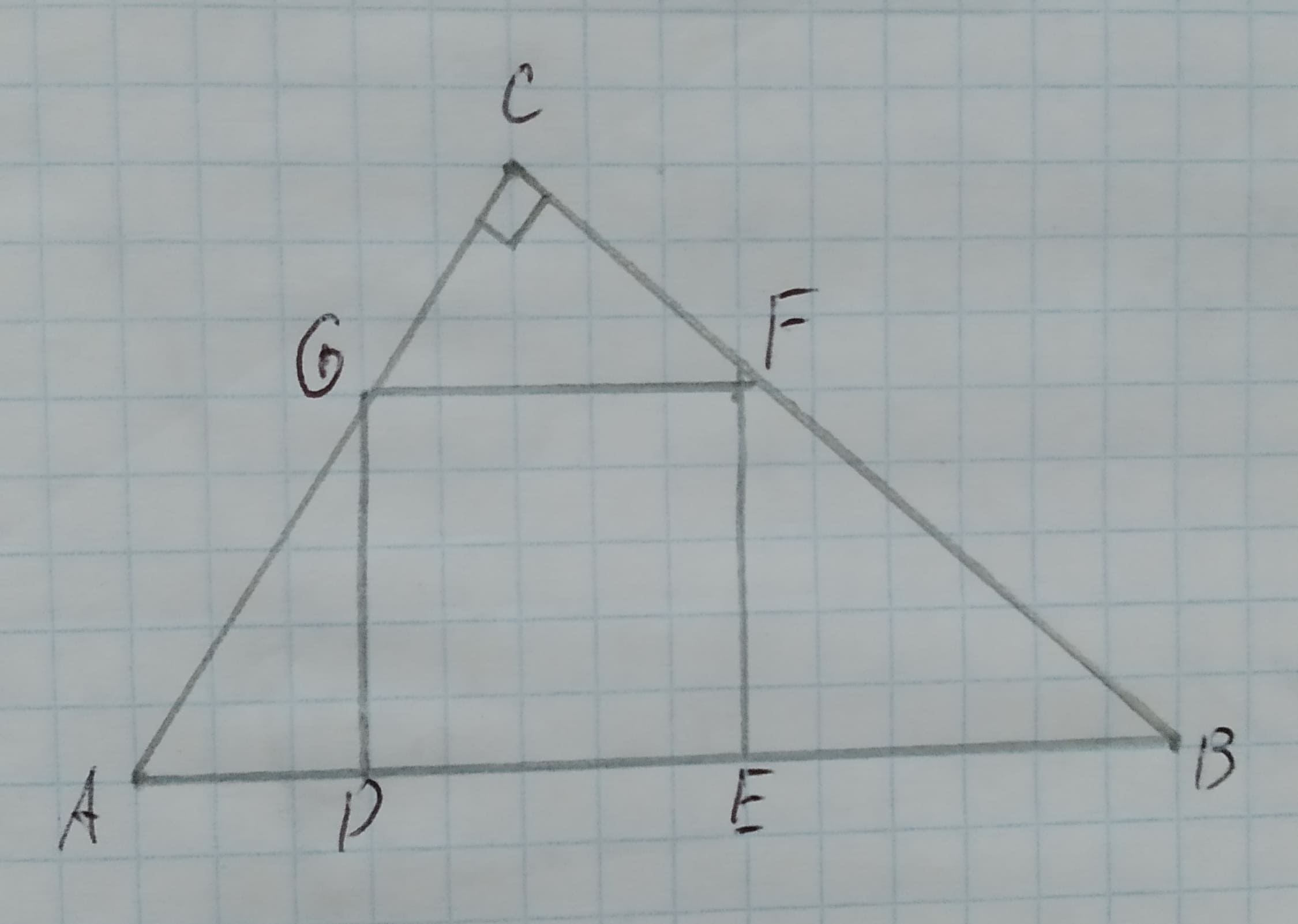# Proving Similarity in the figure DEFG is a square. Prove the following:/_ADG~/_GCFGiven:The given figure is,12210202691.jpgFizeauV 2021-02-02 Answered

Proving Similarity in the figure DEFG is a square. Prove the following:
$\mathrm{△}ADG\sim \mathrm{△}GCF$
Given:
The given figure is,You can still ask an expert for help

• Questions are typically answered in as fast as 30 minutes

Solve your problem for the price of one coffee

• Math expert for every subject
• Pay only if we can solve itMaciej Morrow
Approach:
Two triangles are similar if their vertices can be matched up so that corresponding angles are congruent. In this case corresponding sides are proportional.
If the two angles of the triangles are same then the third angle of the triangles have to be same,because the sum of angles in a triangle is ${180}^{\circ }$. Therefore, the triangles are similar by AA rule if two angles are same.
Calculation:
It is given that DEFG is a square.
Consider $\mathrm{△}ADG\phantom{\rule{1em}{0ex}}\text{and}\phantom{\rule{1em}{0ex}}\mathrm{△}GCF$.
$\mathrm{\angle }ADG=\mathrm{\angle }GCF$ both are ${90}^{\circ }$.
And $GF\mid \mid AB$, thus, $\mathrm{\angle }CGF=\mathrm{\angle }GAD$ as they are alternate angles.
Therefore, $\mathrm{△}ADG\sim \mathrm{△}GCF$ by AA rule.
Conclusion:
Hence, it is proved that $\mathrm{△}ADG\sim \mathrm{△}GCF$.